Your data analysis solution

# Box-Cox transformation tutorial in Excel

2017-10-20

This tutorial shows how to perform a Box-Cox transformation on your data in Excel using the XLSTAT statistical software.

## Dataset for variable transformation

An Excel sheet with both the data and the results can be downloaded by clicking here. In this tutorial we show how to create transform a variable to be closer to the Normal distribution.

The dataset contains the measurements of waste in the production for 47 batches. We would like to make a regression with several process variables but the hypothesis of Normality of the variable Level of waste is not acceptable. We need to make a transformation of this variable before attempting a multilinear regression. After showing you different options to transform data we will use the Box-Cox transformation of XLSTAT.

The results of the Normality test are displayed bellow.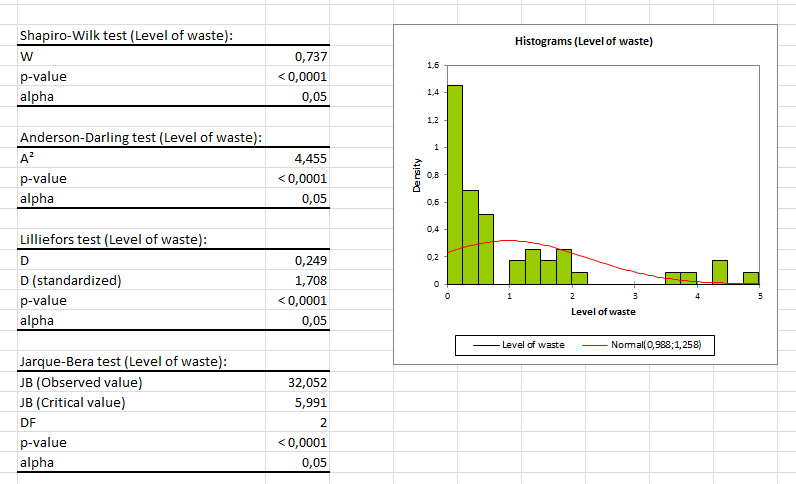## Variable transformation in XLSTAT

There are several ways to transform data in XLSTAT.

### Variable transformation with Microsoft Excel tools

First you can take advantage of Microsoft Excel and use the available function in the software. First place the cursor where you would like to have the results displayed. You will access the menu Insert Function by clicking on the fx icon above the spreadsheet.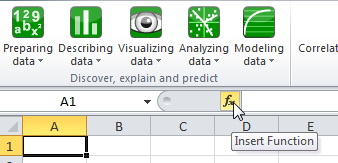Then you can select one of the functions listed under either Financial, Math & Trig, Statistical, Database or XLSTAT (last entry).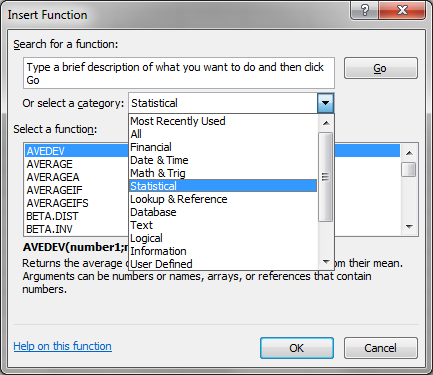This gives you access to a wide range of general transformation.

### Variable transformation with XLSTAT tools

In XLSTAT we offer you the opportunity to use some more specific functions. You will find them in the option Preparing data / Variables transformation.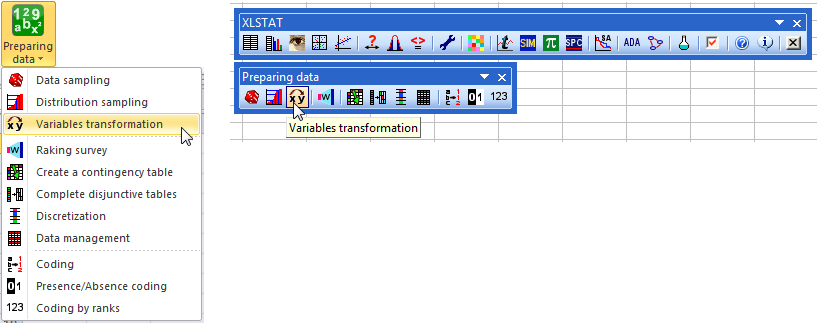## Setting up a Box-Cox transformation

In the dialog box that opens you should first select the variables you wish to transform, in this example we select the variable Level of waste in the column B. Also as the column has a label we tick the option Column labels.

Also we can select the Observation labels option by ticking the box and selecting the column A which contains the identifications of the batches.

The results will be displayed in a new sheet as the option Sheet is selected. If you wish to have them at a specific place select the option Range.

The most general transformation is an unbiased standardization (Standardize (n-1)) as usually people work on a sample and not the full population. However there are more transformations available when you tick the option Other.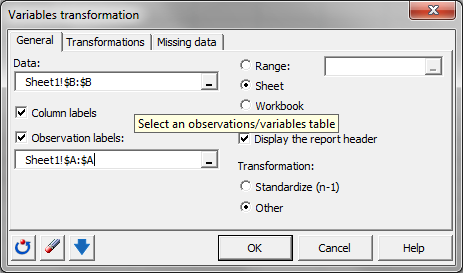Then go on the next tab Transformations that contains the following options:

• Standardize (n): to standardize the variables using the biased standard deviation.
• Center: to center the variables, the average of the resulting variables will be 0.
• 1 / Standard deviation (n-1): to divide the variables by their unbiased standard deviation.
• 1 / Standard deviation (n): to divide the variables by their biased standard deviation.
• Rescale from 0 to 1: to rescale the data from 0 to 1.
• Rescale from 0 to 100: to rescale the data from 0 to 100.
• Binarize (0/1): to convert all values that are not 0 to 1, and leave the 0s unchanged.
• Sign (-1/0/1): to convert all values that are negative to -1, all positive values to 1, and leave the 0s unchanged.
• Arcsin: to transform the data to their arc-sine.
• Box-Cox transformation: to improve the normality of the sample. XLSTAT accepts a fixed value of l, or it can find the value that maximizes the likelihood of the sample, assuming the transformed sample follows a normal distribution.
• Winsorize: to remove data that are not within an interval defined by two percentiles: let p1 and p2 be two values comprised between 0 and 1, such that p1 < p2

Select the option Box-Cox transformation as we are trying to get the variable “Level of waste” closer to a Normal distribution. Also select the option Optimize to let XLSTAT find the best Lambda.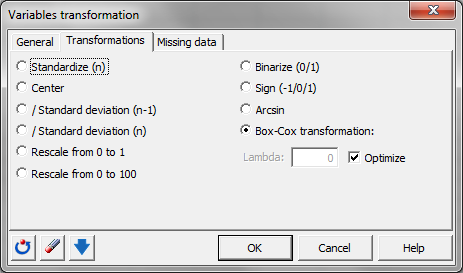The last tab Missing data help you decide what to do in case of missing data. The option selected by default Do not accept missing data will give you a warning in case of missing data. Leave that option selected.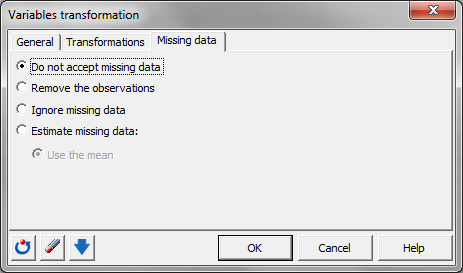Click on OK to start the computations.

## Results of the Box-Cox transformation

In the result sheet called Variables transformation you will find the Transformed data with the value of Lambda used.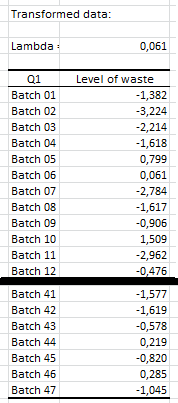You can now compute the Normality test on those transformed data. As you can see bellow now the transformed variable Level of waste is following a Normal distribution.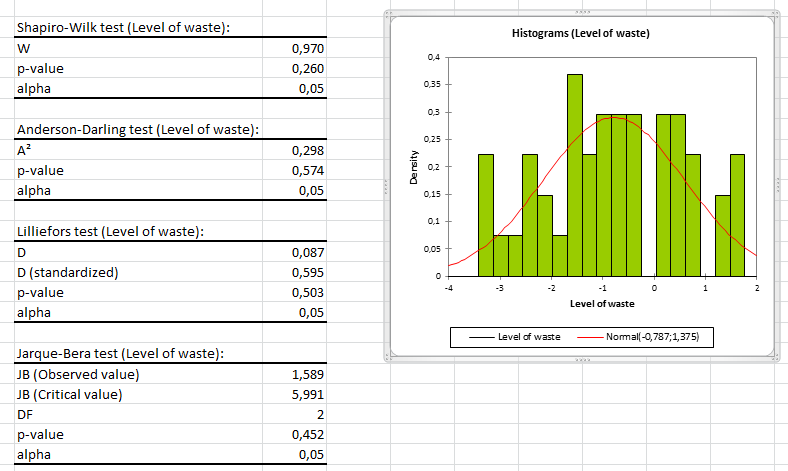### Contact Us

#### Contact our technical support team: support@xlstat.com

https://cdn.desk.com/
false
desk
Loading
seconds ago
a minute ago
minutes ago
an hour ago
hours ago
a day ago
days ago
about
false
Invalid characters found
/customer/portal/articles/autocomplete
9283